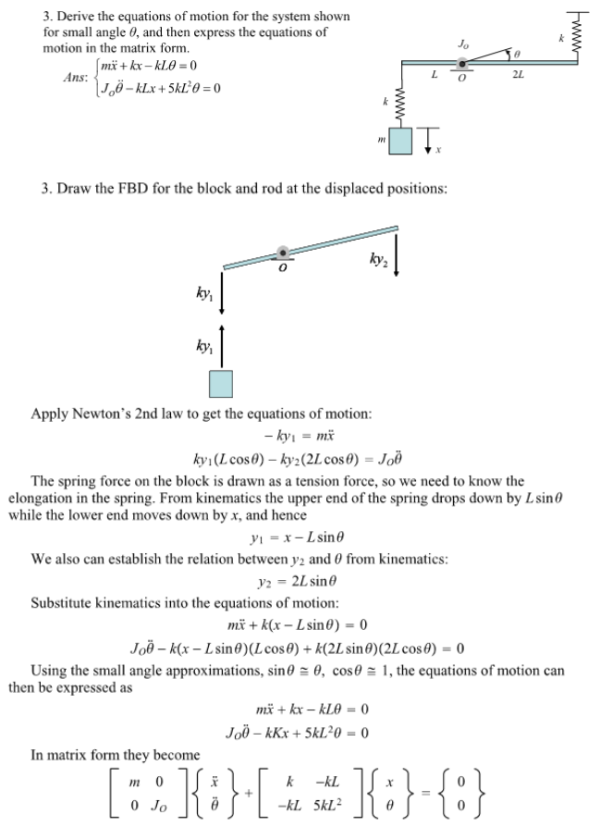Derive the equations of motion for the system shown for small angle theta, and then express the equations of motion in the matrix form.Derive the equations of motion for the system shown for small angle theta, and then express the equations of motion in the matrix form.

System Dynamics Page 2 dynamics dynamics dynamics dynamics dynamics dynamics dynamics System dynamics Page 3